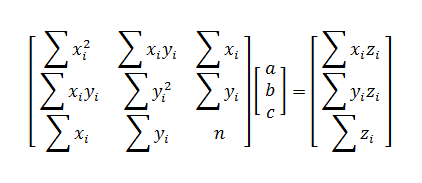# Multiple Linear Regression:z = ax + by + c

Enter X, Y, and Z Data Triples Below
 X Y Z
 X Y Z

#### Least Squares Regression Plane z = ax + by + c

Traditionally, the method of least squares regression allows you to find a two-variable linear equation y = mx + b that provides the "best fit" for the data points. In ordinary least squares, fit is defined as minimizing the squared vertical errors, that is finding the values of m and b that minimize the function

F(m, b) = ∑(yi - mxi - b)².

The solution can be found with matrices since the system ∂F/∂m = 0 and ∂F/∂b = 0 is a linear system of equations.

In multiple linear regression, you can extend the basic idea to find the equation of a plane z = ax + by + c that minimizes the vertical distances between the points (xi, yi, zi) and the plane. To do this, you must find the values of a, b, and c that minimize the equation

G(a, b, c) = ∑(zi - axi - byi - c)²

by solving the system ∂G/∂a = 0, ∂G/∂b = 0, and ∂G/∂c = 0.

#### Solving for a, b, and c

Since the system ∂G/∂a = 0, ∂G/∂b = 0, and ∂G/∂c = 0 is linear, you can solve it with matrices. The matrix equation for a, b, and c isWhen the matrix on the left is invertible (determinant not equal to zero) then there is a unique solution set (a, b, c).

#### Example

A city planning official wants to find a linear function that predicts the length of construction project based on the square footage of the finished building and the size of the construction firm (number of workers). That is, she wants to find a function

z = ax + by + c

where z is the duration of the construction in days, x is the square footage of the finished building, and y is the number of workers. She has the following (x, y, z) data from past building projects:

(10000, 50, 62)   (15000, 120, 45)   (10500, 78, 40)
(25000, 40, 124)   (18000, 85, 68)   (19000, 80, 90)

Using the multiple linear regression calculator above, the least squares plane is

z = 0.0042x - 0.503y + 40.6801.

The coefficients of this equation make sense in the context of the problem. The coefficient of x is a positive number, since the time it takes to complete a project increases as the size of the project increases. The coefficient of y is negative since the more workers there are, the less time it takes to finish the work.

If the city official uses this equation to estimate the duration of a 16500 sq.ft. building project with 95 workers, she plugs x = 16500 and y = 95 into the equation. This yields

z = 0.0042(16500) - 0.503(95) + 40.6801
= 62.1951 days.

The linear regression model may not be the most appropriate model for the data. There are also power regression and exponential regression models for three dimensional data.